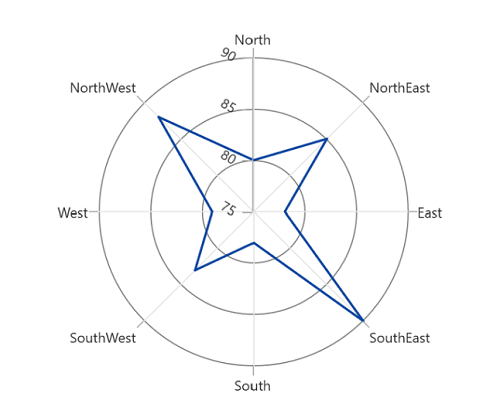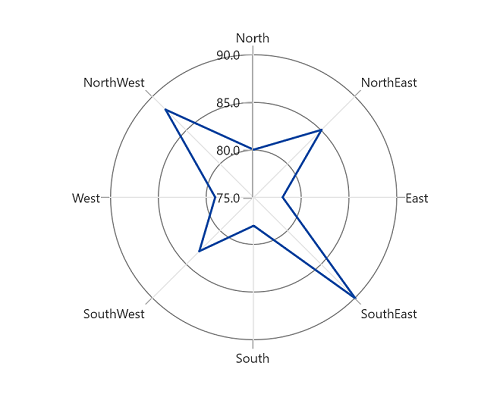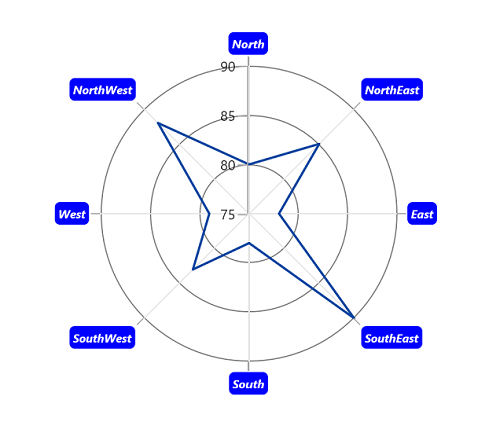# Axis labels in WinUI Chart

29 Jun 20223 minutes to read

The axis labels are used to show the units, measures, or category values of the axis to visualize the data. It will be generated based on the range and values binded to the series in the chart.

## Rotation

The LabelRotationAngle property is used to define the angle for the label content.

``````<chart:SfPolarChart>
...
<chart:SfPolarChart.SecondaryAxis>
<chart:NumericalAxis LabelRotationAngle="30"/>
</chart:SfPolarChart.SecondaryAxis>
...
</chart:SfPolarChart>``````
``````SfPolarChart chart = new SfPolarChart();
...
chart.PrimaryAxis = new CategoryAxis();
chart.SecondaryAxis = new NumericalAxis()
{
LabelRotationAngle = 30,
}
...``````## Format

Axis labels can be formatted by predefined formatting types by using the LabelFormat property based on the axis types.

``````<chart:SfPolarChart>

<chart:SfPolarChart.PrimaryAxis>
<chart:CategoryAxis/>
</chart:SfPolarChart.PrimaryAxis>

<chart:SfPolarChart.SecondaryAxis>
<chart:NumericalAxis LabelFormat="0.0"/>
</chart:SfPolarChart.SecondaryAxis>
...

</chart:SfPolarChart>``````
``````SfPolarChart chart = new SfPolarChart();

chart.PrimaryAxis = new CategoryAxis();

chart.SecondaryAxis = new NumericalAxis()
{
LabelFormat = "0.0",
};
...``````## Template

The appearance of the axis labels can be customized by using the LabelTemplate property of axis.

``````<Grid x:Name="grid">
<Grid.Resources>
<DataTemplate x:Key="labelTemplate">
<TextBlock Text="{Binding Content}" Foreground="White" FontStyle="Italic" FontSize="10" FontWeight="Bold" Margin="3"/>
</Border>
</DataTemplate>
</Grid.Resources>
<chart:SfPolarChart x:Name="chart">
...
<chart:SfPolarChart.PrimaryAxis>
<chart:CategoryAxis LabelTemplate="{StaticResource labelTemplate}"/>
</chart:SfPolarChart.PrimaryAxis>

<chart:SfPolarChart.SecondaryAxis>
<chart:NumericalAxis/>
</chart:SfPolarChart.SecondaryAxis>
...
</chart:SfPolarChart>
</Grid>``````
``````SfPolarChart chart = new SfPolarChart();

chart.PrimaryAxis = new CategoryAxis()
{
LabelTemplate = grid.Resources["labelTemplate"] as DataTemplate
};

chart.SecondaryAxis = new NumericalAxis();
...``````